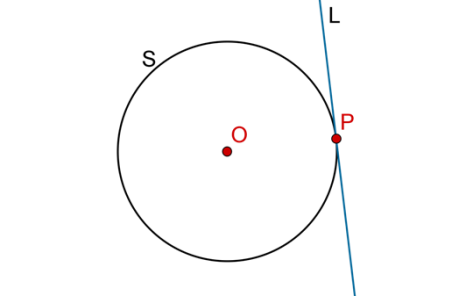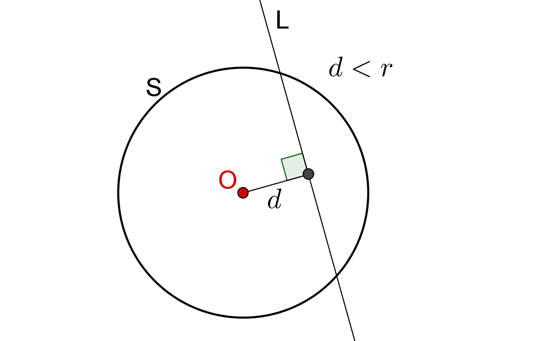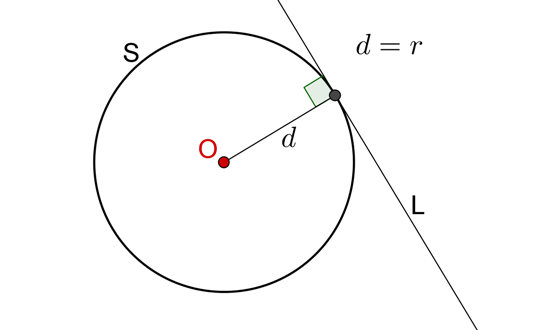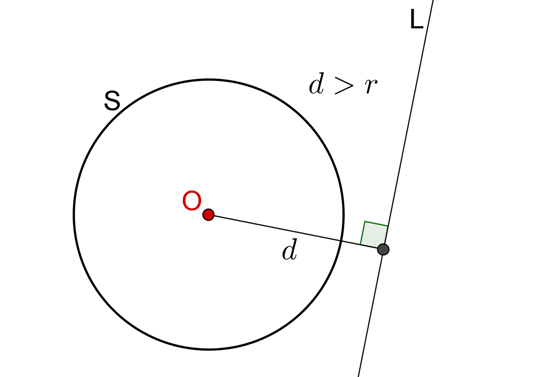# What is a Tangent?

Go back to  'Circles'

We know that a straight line will cut a circle in at the most two points (why cannot it cut a circle in more than two points?). If a line intersects a circle in just one point, it will be said to touch the circle. Such a line will be called a tangent to the circle, and the point at which it touches the circle will be called the point of contact of that tangent. The following figure shows a circle S and one of its tangent L, with the point of contact being P:Can you think of some practical situations which are physical approximations of the concept of tangents? Think, for example, of a very rigid disc rolling on a very flat surface.

A line cutting a circle in two distinct points will be called a secant of that circle. Consider a circle S with center O and radius r, and consider a line L. Let the distance between O and L be d. Given the relation between d and r, we can determine the relation between L and S, as follows:

• If $$d{\rm{ }} < {\rm{ }}r$$ , L is a secant for S:• If $$d{\rm{ }} = {\rm{ }}r$$, L is a tangent to S:• If $$d{\rm{ }} > {\rm{ }}r$$, L is neither a tangent nor a secant for S:The important thing to remember is that the distance of any tangent from the center of the circle is equal to its radius, and the distance of any secant from the center is less than the radius.

Circles and Tangents
grade 10 | Questions Set 1
Circles and Tangents
Circles and Tangents
grade 10 | Questions Set 2
Circles and Tangents
Circles and Tangents
grade 10 | Questions Set 1
Circles and Tangents
Circles and Tangents